Wed, Dec 7, 2005, 5:57pm tex2im brings TeX to this blog
(Last updated: Tue, Mar 14, 2006, 2:39am)
U
sing the very fine tex2im, which corrals the relevant TeX programs on the server (latex, dvips, convert), I can now pop in math equations as images, thusly: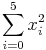$tex image$\sum_{i=0}5 x_i2 and$tex image$f(x) = \sqrt{1+x} \quad (x \ge -1 )

just by typing:
$$\sum_{i=0}5 x_i2$$
$$f(x) = \sqrt{1+x} \quad (x \ge -1 )$$

The first time the page comes up the images are generated on the fly (takes a few seconds), but from then on are available as a regular image. I'll add this feature to the discussion forums too. It will really make the math/physics stuff I want to discuss much better. You just surround your TeX code with $$…$$ tags. (note: I changed this from <tex> tags which I started with.)

I was pulling out my hair there for a while trying to figure out whether PHP was holding me back or permissions or something else, and it was something else: the paths inside of tex2im which pointed to the other scripts had to be given absolute paths. Spun my wheels for a bit there, but it works now.

I was going to present the equations using the transparency option, but the results are not as nice looking, so I just put them in white boxes instead.

• Jeff (Wed, December 7th, 2005, 11:57pm UTC)
Testing user tex comments: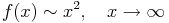$tex image$f(x) \sim x2 , \quad x\to\infty

• Jeff (Thu, December 8th, 2005, 3:12am UTC)
Supposedly, I can change the background color using dvips by setting a flag like -I, but I can't find any decent examples about why my attempt to make this work isn't going anywhere. It'd be nice to have the equation background color match the page.

• Jeff (Thu, December 8th, 2005, 2:28pm UTC)
Ah, much better. Tweaked the background page color code in tex2im to work with the rgb values I need for page design here.

• Jeff (Sun, December 11th, 2005, 1:01pm UTC)
test:$tex image$\displaystyle \pi = \sum _{k=0} ^{\infty} \dfrac{1}{16^k} \left[\dfrac{4}{8k+1}-\dfrac{2}{8k+4}-\dfrac{1}{8k+5}-\dfrac{1}{8k+6}\right]

• Jeff (Sun, December 11th, 2005, 2:17pm UTC)$tex image$\displaystyle\int_{0}^{1}\frac{x^{4}\left(1-x\right)^{4}}{1+x^{2}}dx= \frac{22}{7}-\pi

• Jeff (Tue, April 3rd, 2007, 11:16am UTC)
For those seeing this past the year 2007, the danger of embedding the background color in the equation image is painfully clear once the CSS design is changed. I hope to change the equation system soon to take this into account and some other things. (In fact, if it's changed, you won't even see the color problem I'm referring to in this post.)

• hehe (Wed, June 6th, 2007, 6:04pm UTC)$tex image$$x,f(x),$

• hehe (Wed, June 6th, 2007, 6:05pm UTC)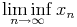$tex image$\liminf_{n \to \infty} x_n

• hehe (Wed, June 6th, 2007, 6:06pm UTC)$tex image$\sum_{i=0}5 x_i2

• Jeff (Wed, June 6th, 2007, 10:43pm UTC)
Hmmm. Just as I was worried about. I updated something on the server recently, and it apparently messed with the TeX system in the blog. I'll have to get to that soon.

• Jeff (Thu, June 7th, 2007, 3:10pm UTC)
Yea! Thanks to some debugging help from John, the TeX system is back and better than before.

• Jeff (Thu, June 7th, 2007, 4:14pm UTC)
One more test of a classic equation: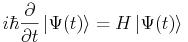$tex image$i\hbar\frac{\partial}{\partial t}\left|\Psi(t)\right>=H\left|\Psi(t)\right>

• Jeff (Mon, September 1st, 2008, 4:40pm UTC)
Test:$tex image$\mathbb{Z}, \mathbb{Q}, \mathbb{R}, \mathbb{C}

• Jeff (Mon, September 1st, 2008, 4:42pm UTC)
Testing more: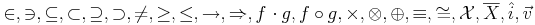$tex image$\in , \ni , \subseteq , \subset , \supseteq , \supset , \ne , \ge , \le , \to , \Rightarrow , f \cdot g , f \circ g , \times , \otimes , \oplus , \equiv , \cong , \mathcal{X} , \overline{X} , \hat{i} , \vec{v}

• dsfdsfd (Tue, April 21st, 2009, 2:46am UTC)$tex image$\sum_{i=0}5 x_i2$tex image$f(x) = \sqrt{1+x} \quad (x \ge -1 )

• savserg (Tue, May 19th, 2009, 8:11am UTC)$tex image$\frac{1}{\sqrt[2 \pi]}\int_{-\infty}^{x}-t2 /2dx

• savserg (Tue, May 19th, 2009, 8:12am UTC)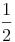$tex image$\frac12

• Jeff (Tue, May 19th, 2009, 11:51am UTC)
savserg, I think the problem was just changing \sqrt[2 \pi] for \sqrt{2 \pi} :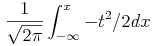$tex image$\frac{1}{\sqrt{2 \pi}}\int_{-\infty}^{x}-t2 /2dx

• Jeff (Tue, March 29th, 2011, 4:17pm UTC)
testing:$tex image$f(x) = \sqrt{1+y3 } \quad (y \ge -1 )

• kingsun (Sun, April 22nd, 2012, 10:06pm UTC)
Test
\frac{1}{\sqrt{2 \pi}}\int_{-\infty}^{x}-t2 /2dx

• kingsun (Sun, April 22nd, 2012, 10:07pm UTC)$tex image$\sum_{i=0}5 x_i2$tex image$f(x) = \sqrt{1+x} \quad (x \ge -1 )

• Jeff (Sun, April 22nd, 2012, 10:14pm UTC)
kingsun, the LaTeX on the current server is a bit broken, but we're about to move servers soon and I hope to re-install LaTeX at that time. Thanks for trying it out at any rate. I should've put a note on this page that it's on temporary hiatus, but it'll be back again soon.